# R S Aggarwal Solutions for Class 11 Maths Chapter 28 Differentiation Exercise 28A

R S Aggarwal Solutions for Class 11 Maths are given here for Chapter 28 exercise 28A. This exercise is based on derivative of a function using some important results on differentiation and first principle. R S Aggarwal class 11 exercise 28A pdf contains solution of all the questions listed in this exercise.

Class 11 Maths Chapter 28 Differentiation Exercise 28A is based on the following topics:

• Derivative of a function

-Derivative at a point

– Geometrical significance of a derivative

-Physical significance of a derivative

• Differentiation from first principle
• Some important derivative results

## Download PDF of R S Aggarwal Solutions for Class 11 Maths Chapter 28 Differentiation Exercise 28A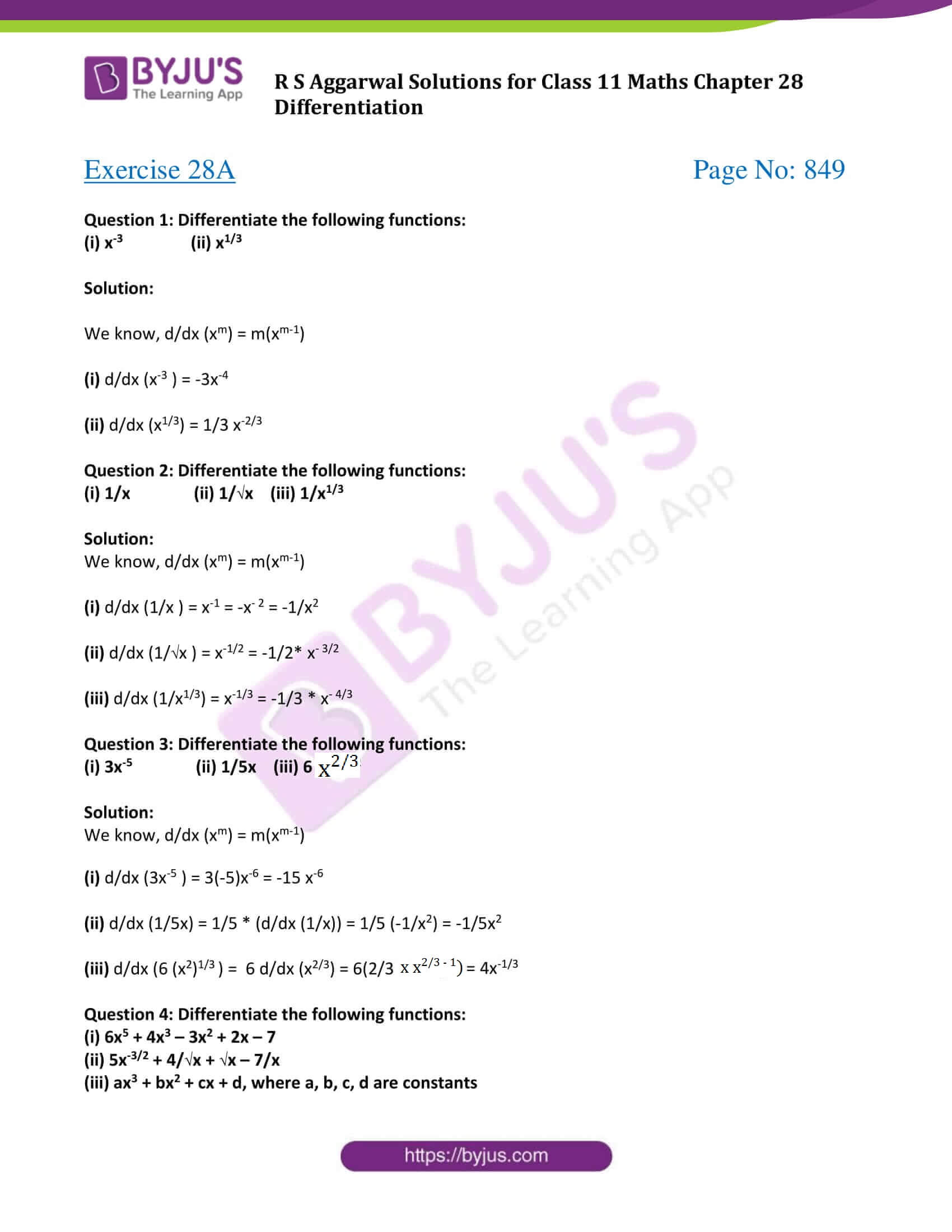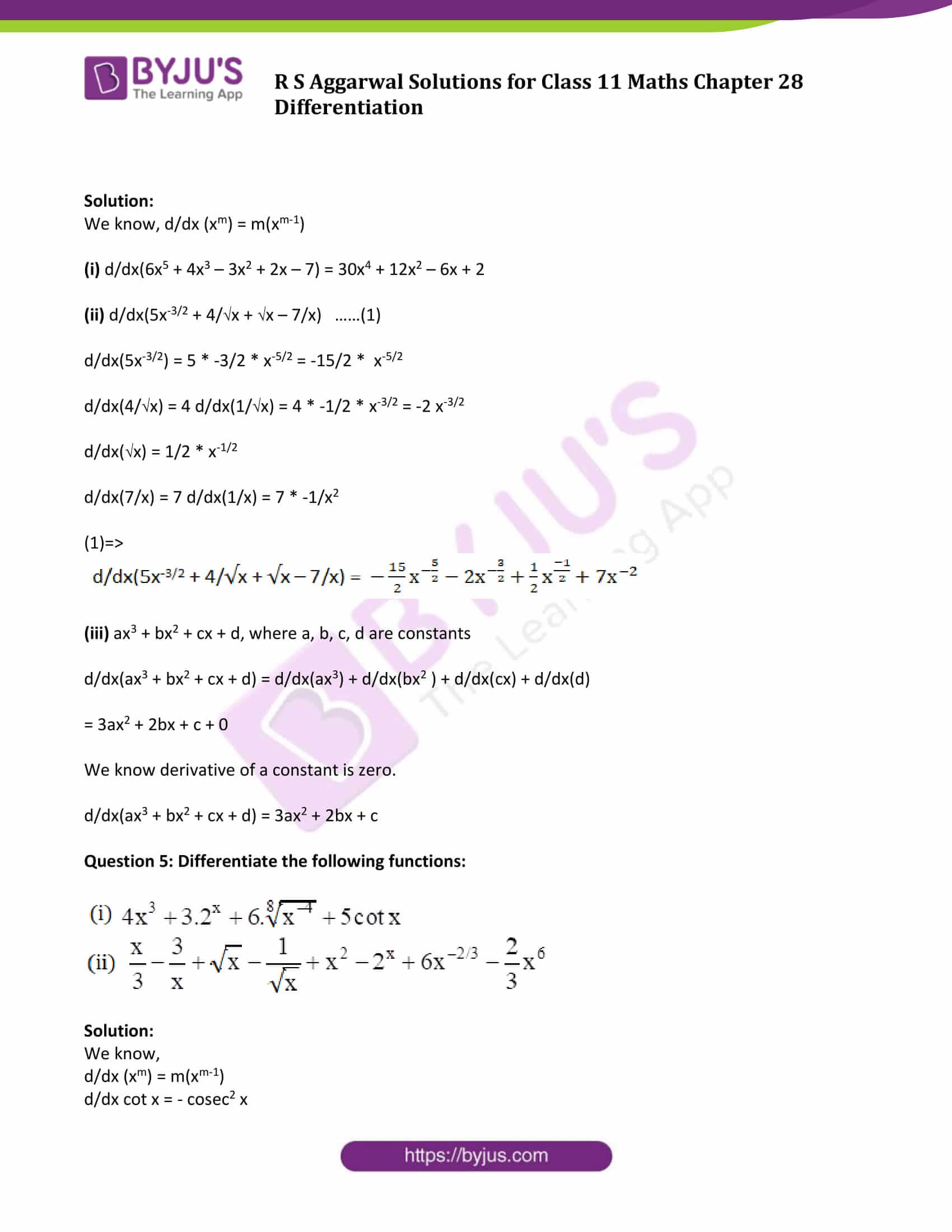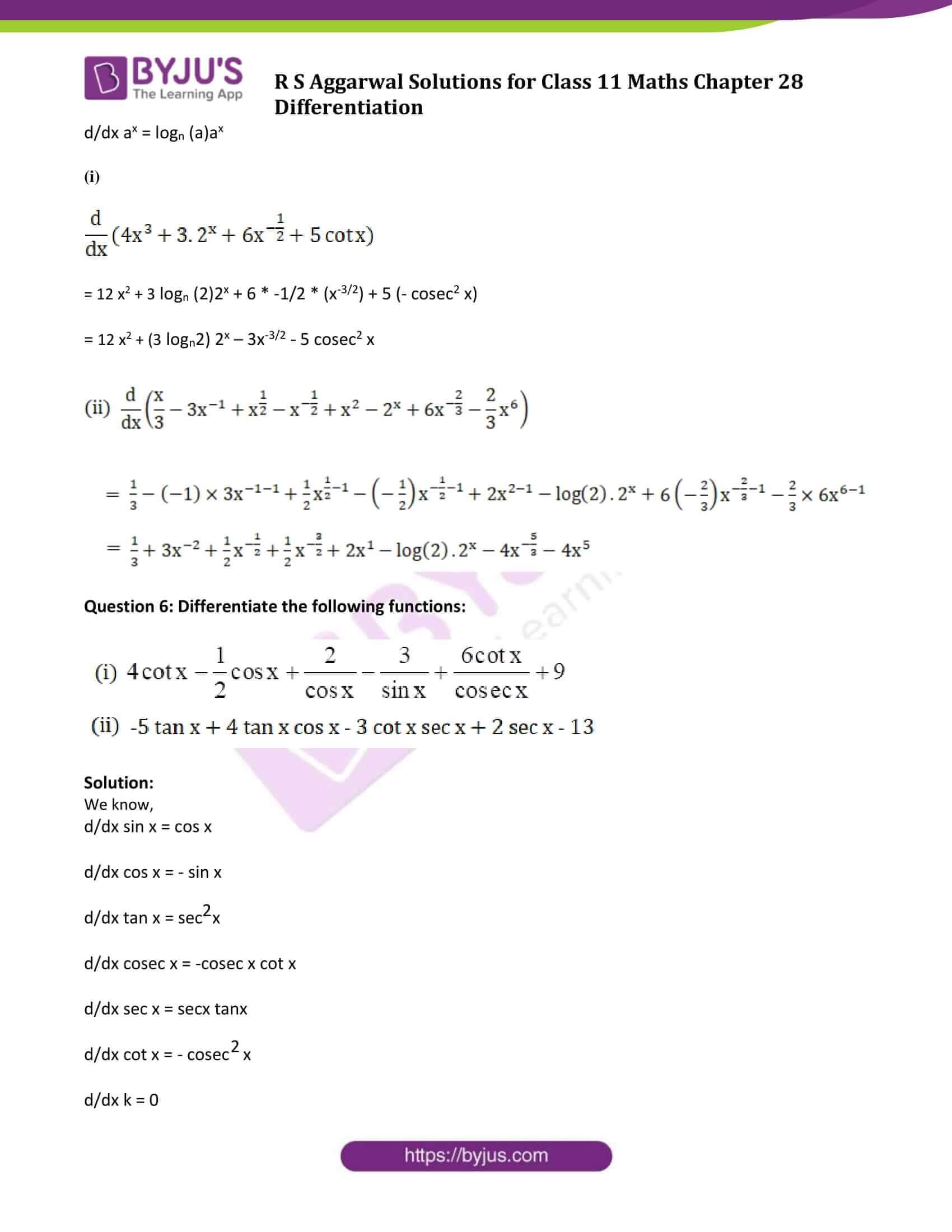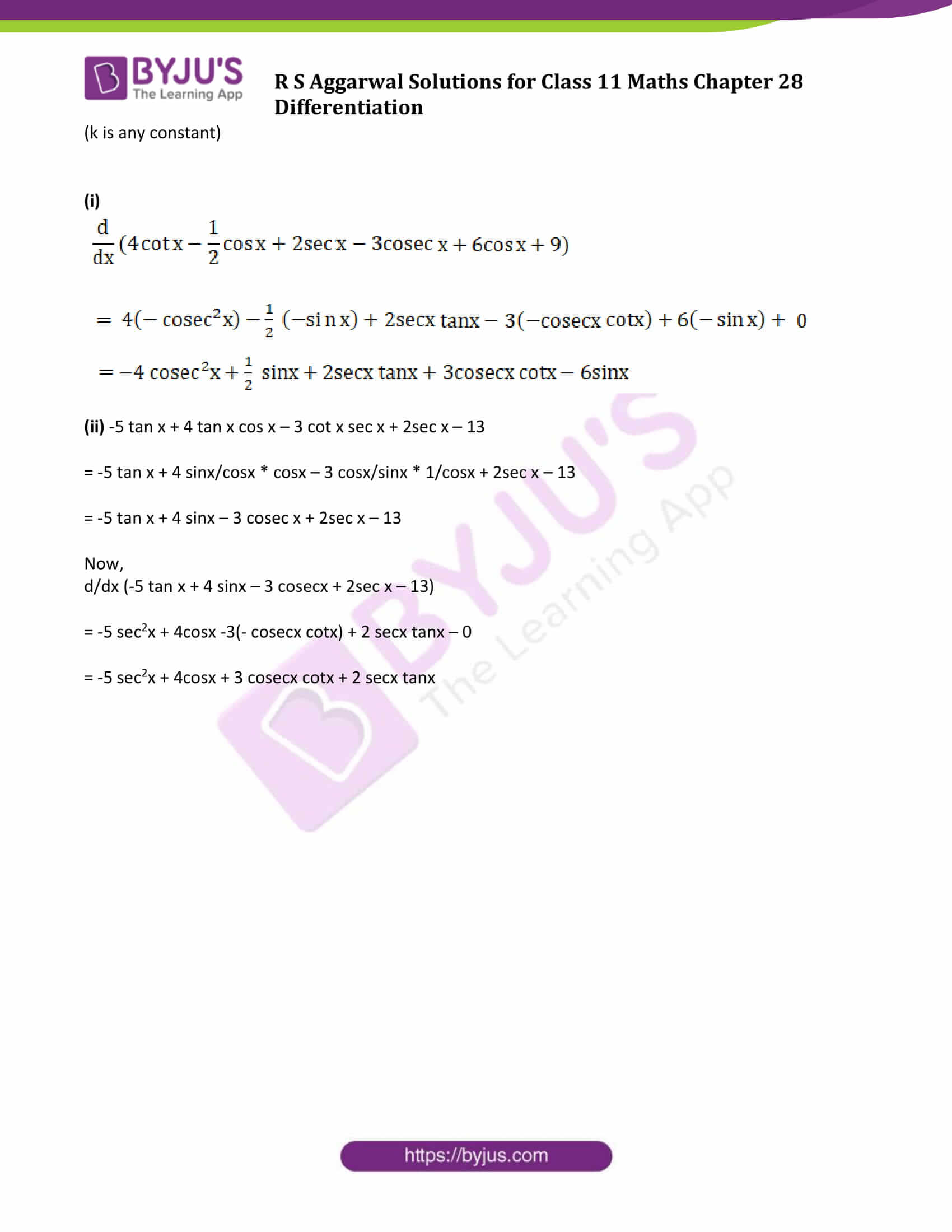### Access Answers to Maths R S Aggarwal Class 11 Chapter 28 Differentiation Exercise 28A Page number 849

Question 1: Differentiate the following functions:

(i) x-3 (ii) x1/3

Solution:

We know, d/dx (xm) = m(xm-1)

(i) d/dx (x-3 ) = -3x-4

(ii) d/dx (x1/3) = 1/3 x-2/3

Question 2: Differentiate the following functions:

(i) 1/x (ii) 1/x (iii) 1/x1/3

Solution:

We know, d/dx (xm) = m(xm-1)

(i) d/dx (1/x ) = x-1 = -x– 2 = -1/x2

(ii) d/dx (1/√x ) = x-1/2 = -1/2* x– 3/2

(iii) d/dx (1/x1/3) = x-1/3 = -1/3 * x– 4/3

Question 3: Differentiate the following functions:

(i) 3x-5 (ii) 1/5x (iii) 6 x2/3

Solution:

We know, d/dx (xm) = m(xm-1)

(i) d/dx (3x-5 ) = 3(-5)x-6 = -15 x-6

(ii) d/dx (1/5x) = 1/5 * (d/dx (1/x)) = 1/5 (-1/x2) = -1/5x2

(iii) d/dx (6 (x2)1/3 ) = 6 d/dx (x2/3) = 6(2/3 x x2/3 -1) = 4x-1/3

Question 4: Differentiate the following functions:

(i) 6x5 + 4x3 – 3x2 + 2x – 7

(ii) 5x-3/2 + 4/√x + √x – 7/x

(iii) ax3 + bx2 + cx + d, where a, b, c, d are constants

Solution:

We know, d/dx (xm) = m(xm-1)

(i) d/dx(6x5 + 4x3 – 3x2 + 2x – 7) = 30x4 + 12x2 – 6x + 2

(ii) d/dx(5x-3/2 + 4/√x + √x – 7/x) ……(1)

d/dx(5x-3/2) = 5 * -3/2 * x-5/2 = -15/2 * x-5/2

d/dx(4/√x) = 4 d/dx(1/√x) = 4 * -1/2 * x-3/2 = -2 x-3/2

d/dx(√x) = 1/2 * x-1/2

d/dx(7/x) = 7 d/dx(1/x) = 7 * -1/x2

(1)=>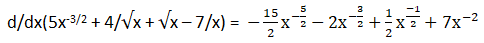(iii) ax3 + bx2 + cx + d, where a, b, c, d are constants

d/dx(ax3 + bx2 + cx + d) = d/dx(ax3) + d/dx(bx2 ) + d/dx(cx) + d/dx(d)

= 3ax2 + 2bx + c + 0

We know derivative of a constant is zero.

d/dx(ax3 + bx2 + cx + d) = 3ax2 + 2bx + c

Question 5: Differentiate the following functions: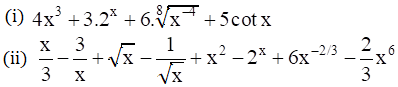Solution:

We know,

d/dx (xm) = m(xm-1)

d/dx cot x = – cosec2 x

d/dx ax = logn (a)ax

(i)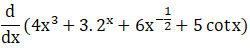= 12 x2 + 3 logn (2)2x + 6 * -1/2 * (x-3/2) + 5 (- cosec2 x)

= 12 x2 + (3 logn2) 2x – 3x-3/2 – 5 cosec2 x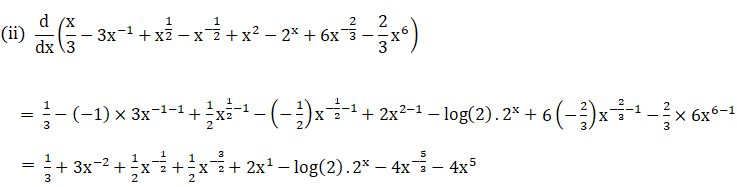Question 6: Differentiate the following functions: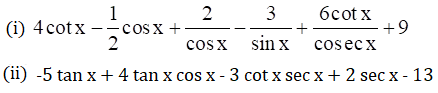Solution:

We know,

d/dx sin x = cos x

d/dx cos x = – sin x

d/dx tan x = sec2x

d/dx cosec x = -cosec x cot x

d/dx sec x = secx tanx

d/dx cot x = – cosec2 x

d/dx k = 0

(k is any constant)

(i)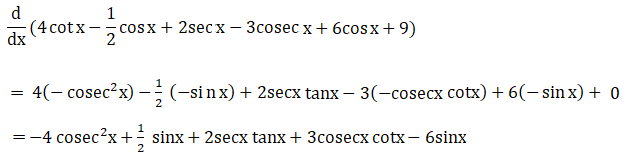(ii) -5 tan x + 4 tan x cos x – 3 cot x sec x + 2sec x – 13

= -5 tan x + 4 sinx/cosx * cosx – 3 cosx/sinx * 1/cosx + 2sec x – 13

= -5 tan x + 4 sinx – 3 cosec x + 2sec x – 13

Now,

d/dx (-5 tan x + 4 sinx – 3 cosecx + 2sec x – 13)

= -5 sec2x + 4cosx -3(- cosecx cotx) + 2 secx tanx – 0

= -5 sec2x + 4cosx + 3 cosecx cotx + 2 secx tanx

## Access other exercise solutions of Class 11 Maths Chapter 28 Differentiation

Exercise 28B Solutions

Exercise 28C Solutions

Exercise 28D Solutions

Exercise 28E Solutions